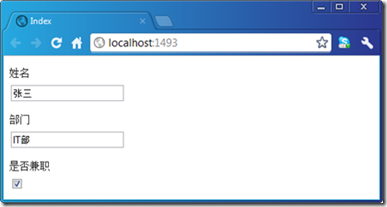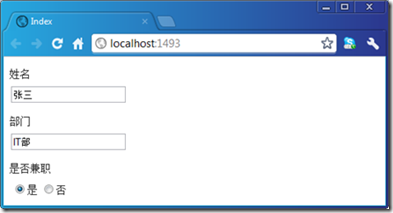## ASP.NET MVC的Model元数据与Model模板：预定义模板

EmailAddress
HiddenInput
Html
Text与String
Url
MultilineText
Password
Decimal
Boolean
Collection
Object

# 一、 实例演示：通过模板将布尔值显示为RadioButton

`   1: public class Employee`
`   2: {`
`   3:     [DisplayName("姓名")]`
`   4:     public string Name { get; set; }`
`   5:  `
`   6:     [DisplayName("部门")]`
`   7:     public string Department { get; set; }`
`   8:  `
`   9:     [DisplayName("是否兼职")]`
`  10:     public bool IsPartTime { get; set; }`
`  11: }``   1: @model bool`
`   2: <table>`
`   3:     <tr>`
`   4:         <td>@Html.RadioButton("",true,Model)是</td>`
`   5:         <td>@Html.RadioButton("",false,!Model)否</td>`
`   6:     </tr>`
`   7: </table>`

`   1: <div class="editor-label"><label for="IsPartTime">是否兼职</label></div>`
`   2: <div class="editor-field">`
`   3:     <table>`
`   4:         <tr>`
`   5:             <td><input checked="checked" id="IsPartTime" name="IsPartTime" type="radio" value="True" .../>是</td>`
`   6:             <td><input id="IsPartTime" name="IsPartTime" type="radio" value="False" />否</td>`
`   7:         </tr>`
`   8:     </table>`
`   9: </div>`

# 二、预定义模板

## EmailAddress

`   1: public class Model`
`   2: {`
`   3:     [UIHint("EmailAddress")]`
`   4:     public string Foo { get; set; }`
`   5: }`

`   1: @model Model`
`   2: @Html.DisplayFor(m=>m.Foo)`

`   1: <a href="mailto:foo@gmail.com">foo@gmail.com</a>`

## HiddenInput

`   1: public class Model`
`   2: {`
`   3:     [UIHint("HiddenInput")]`
`   4:     public string Foo { get; set; }`
`   5:     public bool Bar { get; set; }`
`   6:     public decimal Baz { get; set; }`
`   7: }`

`   1: @model Model`
`   2: @Html.DisplayFor(m=>m.Foo)`
`   3: @Html.EditorFor(m=>m.Foo)`

`   1: {42A1E9B7-2AED-4C8E-AB55-78813FC8C233} `
`   2: {42A1E9B7-2AED-4C8E-AB55-78813FC8C233}<input id="Foo" name="Foo" type="hidden" value="{42A1E9B7-2AED-4C8E-AB55-78813FC8C233}" />`

`   1: public class Model`
`   2: {`
`   3:     [HiddenInput(DisplayValue = false)]`
`   4:     public string Foo { get; set; }`
`   5:     public bool Bar { get; set; }`
`   6:     public decimal Baz { get; set; }`
`   7: }`

`   1: <input id="Foo" name="Foo" type="hidden" value="{42A1E9B7-2AED-4C8E-AB55-78813FC8C233}" />`

## Html

`   1: public class Model`
`   2: {`
`   3:     [UIHint("Html")]`
`   4:     public string Foo { get; set; }`
`   5:     public string Bar { get; set; }`
`   6: }`

`   1: @model Model`
`   2: @Html.DisplayFor(m=>m.Foo)`
`   3: @Html.DisplayFor(m => m.Bar)`

`   1: <a href="www.google.com">google.com</a> &lt;a href=&quot;www.google.com&quot;&gt;google.com&lt;/a&gt;`

## Text与String

`   1: public class Model`
`   2: {`
`   3:     [UIHint("String")]`
`   4:     public string Foo { get; set; }`
`   5:     [UIHint("Text")]`
`   6:     public string Bar { get; set; }`
`   7: }`

`   1: @model Model`
`   2: @Html.DisplayFor(m=>m.Foo)`
`   3: @Html.DisplayFor(m => m.Bar)`
`   4: @Html.EditorFor(m=>m.Foo)`
`   5: @Html.EditorFor(m => m.Bar)`

`   1: Dummy text ... `
`   2: Dummy text ... `
`   3: <input class="text-box single-line" id="Foo" name="Foo" type="text" value="Dummy text ..." /> `
`   4: <input class="text-box single-line" id="Bar" name="Bar" type="text" value="Dummy text ..." />`

## Url

`   1: public class Model`
`   2: {`
`   3:     [UIHint("Url")]`
`   4:     public string Foo { get; set; }`
`   5: }`

`   1: @model Model`
`   2: @Html.DisplayFor(m=>m.Foo)`

`   1: <a href="http://www.asp.net">http://www.asp.net</a>`

## MultilineText

`   1: public class Model`
`   2: {`
`   3:     [UIHint("MultilineText")]`
`   4:     public string Foo { get; set; }`
`   5: }`

`   1: @model Model`
`   2: @Html.EditorFor(m=>m.Foo)`

`   1: <textarea class="text-box multi-line" id="Foo" name="Foo">dummy text ...</textarea>`

## Password

`   1: public class Model`
`   2: {`
`   3:     [UIHint("Password")]`
`   4:     public string Foo { get; set; }`
`   5: }`

`   1: @model Model`
`   2: @Html.EditorFor(m=>m.Foo)`

`   1: <input class="text-box single-line password" id="Foo" name="Foo" type="password" value="" />`

## Decimal

`   1: public class Model`
`   2: {`
`   3:     [UIHint("Decimal")]`
`   4:     public object Foo { get; set; }`
`   5:  `
`   6:     [UIHint("Decimal")]`
`   7:     public object Bar { get; set; }`
`   8: }`

`   1: @model Model`
`   2: @Html.DisplayFor(m=>m.Foo)`
`   3: @Html.DisplayFor(m=>m.Bar)`
`   4: @Html.EditorFor(m=>m.Foo)`
`   5: @Html.EditorFor(m =>m.Bar)`

`   1: 123.00`
`   2: 3.14`
`   3: <input class="text-box single-line" id="Foo" name="Foo" type="text" value="123.00" />`
`   4: <input class="text-box single-line" id="Bar" name="Bar" type="text" value="3.14" />`

## Boolean

`   1: <input id="Foo" name="Foo" type="checkbox" value="true" />`
`   2: <input name="Foo" type="hidden" value="false" />`

Boolean和String、Decimal以及后面我们介绍的Object一样属于是基于CLR类型的模板。由于ASP.NET在内部采用基于类型的模板匹配策略，如果没有显示设置采用的模板类型，相应类型的元素会默认采用与之匹配的模板。

## Collection

`   1: public class Model`
`   2: {`
`   3:     [UIHint("Collection")]`
`   4:     public object[] Foo { get; set; }`
`   5: }`

`   1: object[] foo = new object[]`
`   2: {`
`   3:     123.00,`
`   4:     "dummy text ...",`
`   5:     true`
`   6: };`
`   7: Model model = new Model { Foo = foo };`

`   1: @model Model`
`   2: @Html.DisplayFor(m=>m.Foo)`
`   3: @Html.EditorFor(m=>m.Foo)`

Model对象的Foo属性最终呈现出来的HTML如下所示，我们可以看到不论是显示模式还是编辑模式，基本上就是对集合元素呈现的HTML的组合而已。

`   1: 123dummy text ...<input checked="checked" class="check-box" disabled="disabled" type="checkbox" />`
`   2: <input class="text-box single-line" data-val="true" data-val-number="The field Double must be a number." data-val-required="The Double field is required." id="Foo_0_" name="Foo" type="text" value="123" />`
`   3: <input class="text-box single-line" id="Foo_1_" name="Foo" type="text" value="dummy text ..." />`
`   4: <input checked="checked" class="check-box" data-val="true" data-val-required="The Boolean field is required." id="Foo_2_" name="Foo" type="checkbox" value="true" />`
`   5: <input name="Foo" type="hidden" value="false" />`

## Object

`   1: public class Address`
`   2: {`
`   3:     [DisplayName("省")]`
`   4:     public string Province { get; set; }`
`   5:     [DisplayName("市")]`
`   6:     public string City { get; set; }`
`   7:     [DisplayName("区")]`
`   8:     public string District { get; set; }`
`   9:     [DisplayName("街道")]`
`  10:     public string Street { get; set; }`
`  11: }`

`   1: @model Address`
`   2: @Html.DisplayForModel()`

`   1: <div class="display-label">省</div> `
`   2: <div class="display-field">江苏省</div> `
`   3: <div class="display-label">市</div> `
`   4: <div class="display-field">苏州市</div> `
`   5: <div class="display-label">区</div> `
`   6: <div class="display-field">工业园区</div> `
`   7: <div class="display-label">街道</div> `
`   8: <div class="display-field">星湖街328号</div> `

`   1: public class Contact`
`   2: {`
`   3:     [DisplayName("姓名")]`
`   4:     public string Name { get; set; }`
`   5:     [DisplayName("电话")]`
`   6:     public string PhoneNo { get; set; }`
`   7:     [DisplayName("Email地址")]`
`   8:     public string EmailAddress { get; set; }`
`   9:     [DisplayName("联系地址")]`
`  10:     public Address Address { get; set; }`
`  11: }`

`   1: @model Contact`
`   2: @Html.DisplayForModel()`

`   1: <div class="display-label">姓名</div>`
`   2: <div class="display-field">张三</div>`
`   3: <div class="display-label">电话</div>`
`   4: <div class="display-field">1234567890</div>`
`   5: <div class="display-label">Email地址</div>`
`   6: <div class="display-field">zhangsan@gmail.com</div>`

`   1: @model Contact`
`   2: @Html.DisplayForModel()`
`   3: <div class="display-label">@Html.DisplayName("Address")</div>`
`   4: <div class="display-field">@(Model.Address.Province + Model.Address.City + Model.Address.District+ Model.Address.Street)</div>`